StatLect

# Poisson distribution

The Poisson distribution is a discrete probability distribution used to model the number of occurrences of a random event.## How the distribution is used

Suppose that an event can occur several times within a given unit of time.

When the total number of occurrences of the event is unknown, we can think of it as a random variable.

This random variable has a Poisson distribution if the time elapsed between two successive occurrences of the event:

1. has an exponential distribution;

2. it is independent of previous occurrences.

## Example

A classical example of a random variable having a Poisson distribution is the number of phone calls received by a call center.

If the time elapsed between two successive phone calls has an exponential distribution and it is independent of the time of arrival of the previous calls, then the total number of calls received in one hour has a Poisson distribution.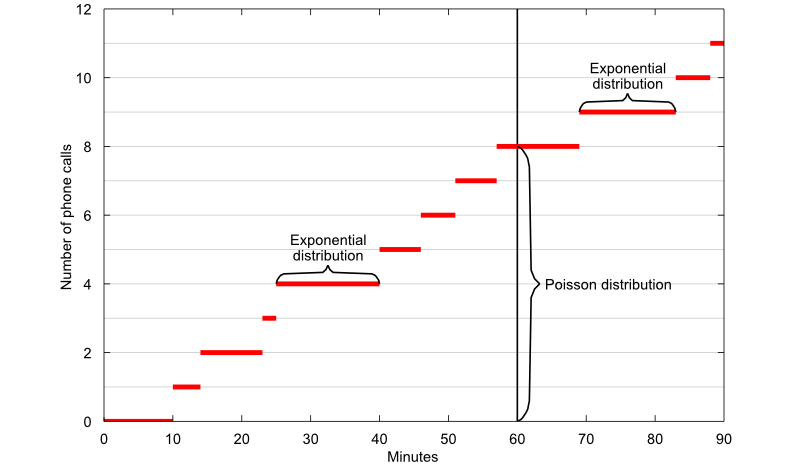The concept is illustrated by the plot above, where the number of phone calls received is plotted as a function of time:

• the graph of the function makes an upward jump each time a phone call arrives;

• the time elapsed between two successive phone calls is equal to the length of each horizontal segment and it has an exponential distribution;

• the number of calls received in 60 minutes is equal to the length of the segment highlighted by the vertical curly brace and it has a Poisson distribution.

## Definition

A Poisson random variable is characterized as follows.

Definition Letbe a discrete random variable. Let its support be the set of non-negative integer numbers:Let. We say thathas a Poisson distribution with parameterif its probability mass function iswhereis the factorial of.

## Relation to the exponential distribution

The relation between the Poisson distribution and the exponential distribution is summarized by the following proposition.

Proposition The number of occurrences of an event within a unit of time has a Poisson distribution with parameterif the time elapsed between two successive occurrences of the event has an exponential distribution with parameterand it is independent of previous occurrences.

Proof

Denote bythe number of occurrences of the event and by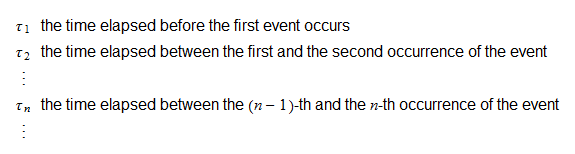Note that there are at leastoccurrences of the event (i.e.,) within a unit of time if and only if the sum of the times elapsed between theoccurrences is less than one unit of time. In other words, the eventsandcoincide. Therefore,for any. Thus, the distribution ofcan be derived from the distribution of the waiting times. We are going to prove that the assumption that the waiting times are exponential implies thathas a Poisson distribution. Denote bythe sum of waiting times:Since the sum of independent exponential random variables with common parameteris a Gamma random variable with parametersand, thenis a Gamma random variable with parametersand, i.e., its probability density function iswhereand the last equality stems from the fact that we are considering only integer values of. We need to integrate the density function to compute the probability thatis less than:The last integral can be computed integrating by partstimes: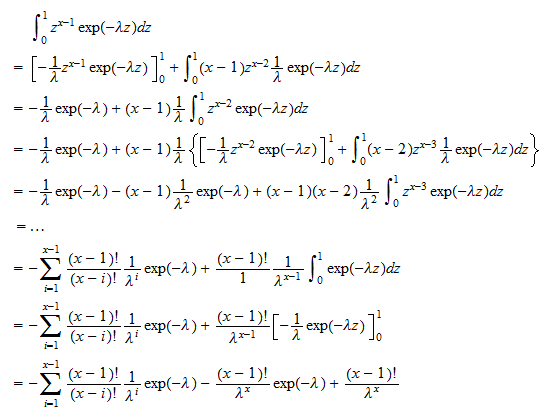Multiplying by, we obtainThus, we have obtained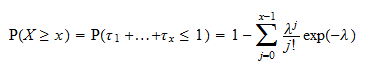But this is exactly what we get whenhas a Poisson distribution:## Expected value

The expected value of a Poisson random variableisProof

It can be derived as follows: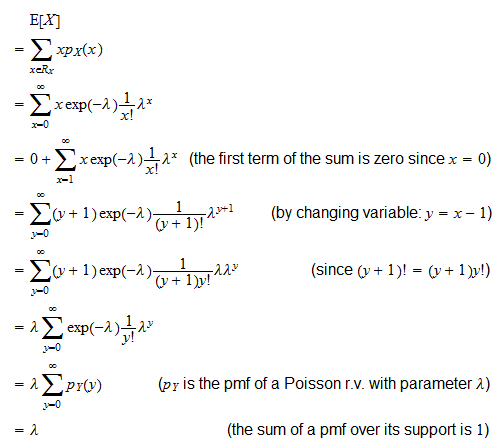## Variance

The variance of a Poisson random variableisProof

It can be derived thanks to the usual variance formula ():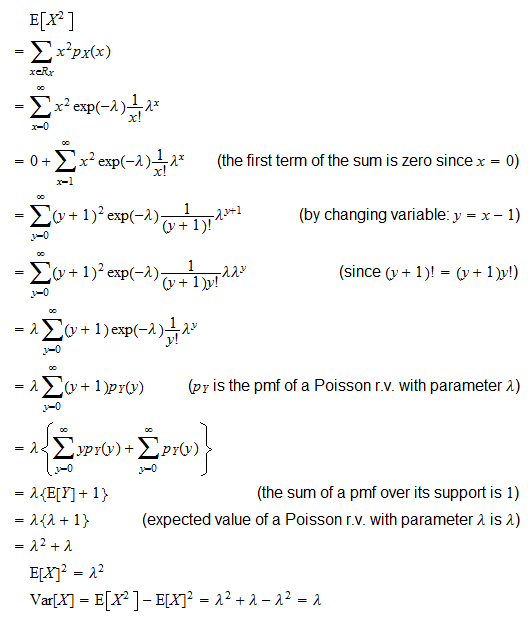## Moment generating function

The moment generating function of a Poisson random variableis defined for any:Proof

By using the definition of moment generating function, we getwhereis the usual Taylor series expansion of the exponential function. Furthermore, since the series converges for any value of, the moment generating function of a Poisson random variable exists for any.

## Characteristic function

The characteristic function of a Poisson random variableisProof

By using the definition of characteristic function, we obtainwhereis the usual Taylor series expansion of the exponential function (note that the series converges for any value of).

## Distribution function

The distribution function of a Poisson random variableiswhereis the floor of, i.e. the largest integer not greater than.

Proof

By using the definition of distribution function, we get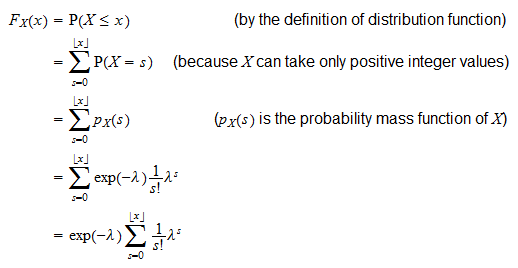Values ofare usually computed by computer algorithms. For example, the MATLAB command:

`poisscdf(x,lambda)`

returns the value of the distribution function at the point `x` when the parameter of the distribution is equal to `lambda`.

## Solved exercises

Below you can find some exercises with explained solutions.

### Exercise 1

The time elapsed between the arrival of a customer at a shop and the arrival of the next customer has an exponential distribution with expected value equal to 15 minutes. Furthermore, it is independent of previous arrivals.

What is the probability that more than 6 customers arrive at the shop during the next hour?

Solution

If a random variable has an exponential distribution with parameter, then its expected value is equal to. HereTherefore,. If inter-arrival times are independent exponential random variables with parameter, then the number of arrivals during a unit of time has a Poisson distribution with parameter. Thus, the number of customers that will arrive at the shop during the next hour (denote it by) is a Poisson random variable with parameter. The probability that more than 6 customers arrive at the shop during the next hour is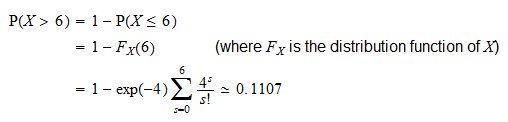and the value ofcan be calculated with a computer algorithm, for example with the MATLAB command### Exercise 2

At a call center, the time elapsed between the arrival of a phone call and the arrival of the next phone call has an exponential distribution with expected value equal to 15 seconds. Furthermore, it is independent of previous arrivals.

What is the probability that less than 50 phone calls arrive during the next 15 minutes?

Solution

If a random variable has an exponential distribution with parameter, then its expected value is equal to. Herewhere, in the last equality, we have taken 15 minutes as the unit of time. Therefore,. If inter-arrival times are independent exponential random variables with parameter, then the number of arrivals during a unit of time has a Poisson distribution with parameter. Thus, the number of phone calls that will arrive during the next 15 minutes (denote it by) is a Poisson random variable with parameter. The probability that less than 50 phone calls arrive during the next 15 minutes is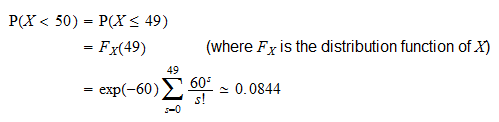and the value ofcan be calculated with a computer algorithm, for example with the MATLAB command

`poisscdf(49,60)`